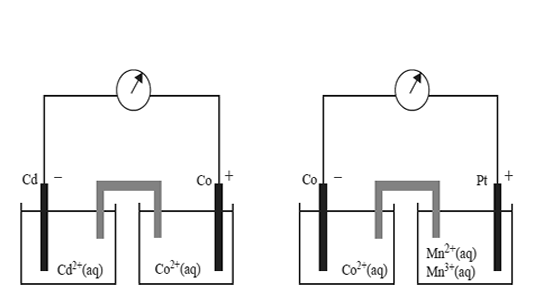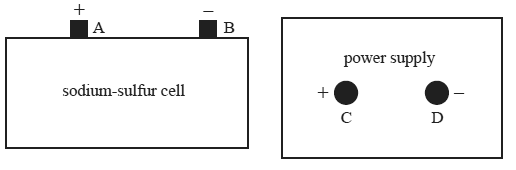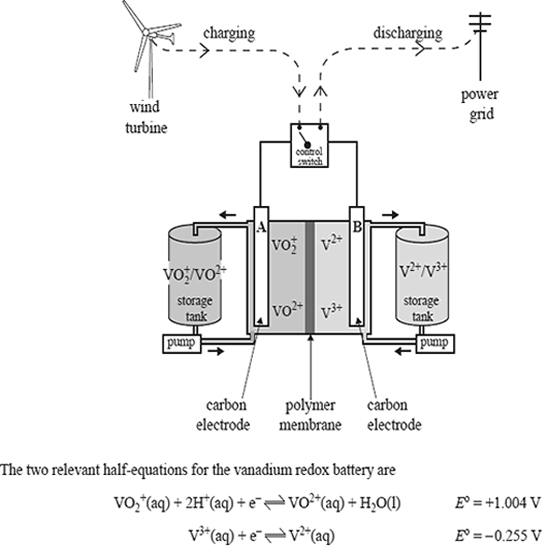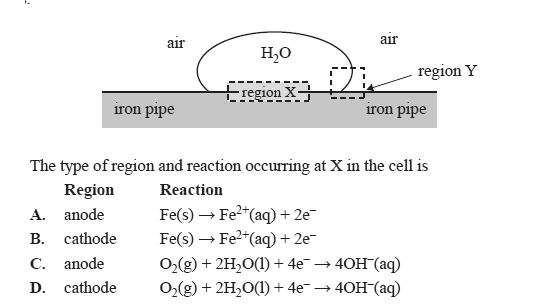Galvanic cells 2009 VCE Two standard galvanic cells are shown below.On the basis of the polarity of the electrodes shown above, which one of the following reactions would not be expected to occur spontaneously? A. Co2+(aq) + Cd(s) → Co(s) + Cd2+(aq) B. 2Mn3+(aq) + Co(s) → 2Mn2<+(aq) + Co2+(aq) C. 2Mn3+(aq) + Cd(s) → 2Mn2+(aq) + Cd2+(aq) D. 2Mn2+(aq) + Co2+(aq) → 2Mn3+(aq) + Co(s) Solution The sodium-sulfur cell shown below is a secondary galvanic cell with the overall cell reaction 2Na(l) + S(l) <=> Na2S(l) The cell produces 2.1 volts.The cell is to be recharged by connecting it to the power supply. Which one of the following best describes the arrangement for recharging the cell? A. Power supply voltage=2.1 volts, connect terminals A to C and B to D B. Power supply voltage=2.1 volts, connect terminals A to D and B to C C. Power supply voltage=more than 2.1, connect terminals volts A to C and B to D D.Power supply voltage= more than 2.1 volts, connect terminals A to D and B to C SolutionState the polarity of each electrode as the battery is discharged. Electrode A Electrode B Solution Circle the vanadium-containing ion that would have the highest concentration at the anode when the cell is fully charged. VO2+, VO2+ , V3+ , V2+ Solution Write a balanced overall equation for the reaction that occurs when the cell is being recharged. Solution Compare the vanadium redox cell to a fuel cell by describing one major way in which they differ. Solution Write a balanced overall equation to show why iron would be an unsuitable material to use as electrode B in the vanadium redox cell. SolutionIron pipes are used to transport natural gas to cities. Corrosion occurs when water droplets sit on the outer surface of the iron pipe. Miniature galvanic cells are created, with regions such as those shown below, that act as anodes and cathodes.Solution